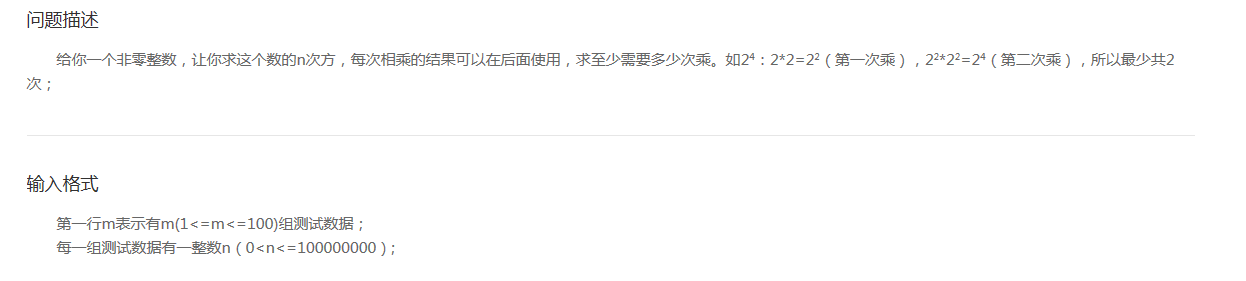# 乘法次数：二分法

问题描述

给你一个非零整数，让你求这个数的n次方，每次相乘的结果可以在后面使用，求至少需要多少次乘。如24：2*2=22（第一次乘），22*22=24（第二次乘），所以最少共2次；

第一行m表示有m(1<=m<=100)组测试数据；
每一组测试数据有一整数n（0<n<=100000000）;

输出每组测试数据所需次数s;

3
2
3
4

1
2
2import java.util.ArrayList;
import java.util.Scanner;

public class 乘法次数 {

public static void main(String[] args) {
// TODO Auto-generated method stub
Scanner sc=new Scanner(System.in);
int m=sc.nextInt();
ArrayList<Integer>input =new ArrayList<Integer>();
ArrayList<Integer>output =new ArrayList<Integer>();
for(int i=0;i<m;i++){
}
for(int i:input){
int temp=0;
while(i!=1){
if(i%2==0)
temp+=1;
else{
temp+=2;
}
i/=2;
}
}
for(int i:output)
System.out.println(i);
}

}©️2019 CSDN 皮肤主题: 技术黑板 设计师: CSDN官方博客